•5星
101KB qq_40957277 2021-09-07 22:51:15
•5星
111.52MB qq_28239511 2021-03-17 13:04:53
•5星
9.55MB HUANGliang_ 2021-08-13 14:52:28
•5星
1.18MB weixin_45771864 2021-07-19 16:11:07
•5星
93.95MB qq_21548021 2021-06-28 10:17:26
•5星
406.57MB qq_42324086 2021-08-05 16:13:28
•5星
139KB qq_20232875 2021-01-08 15:29:56
•5星
5.3MB m0_52384018 2021-07-01 01:59:37
•5星
157KB qq_41934573 2021-05-21 23:17:41
•5星
13KB weixin_37581211 2021-03-28 08:31:57
• python如何实现逻辑回归？本篇文章小编给大家分享一下python实现逻辑回归代码示例，文章代码介绍的很详细，小编觉得挺不错的，现在分享给大家供大家参考，有需要的小伙伴们可以来看看。代码如下：import numpy as np...

python如何实现逻辑回归？本篇文章小编给大家分享一下python实现逻辑回归代码示例，文章代码介绍的很详细，小编觉得挺不错的，现在分享给大家供大家参考，有需要的小伙伴们可以来看看。

代码如下：

import numpy as np

import matplotlib.pyplot as plt

from sklearn.datasets.samples_generator import make_classification

def initialize_params(dims):

w = np.zeros((dims, 1))

b = 0

return w, b

def sigmoid(x):

z = 1 / (1 + np.exp(-x))

return z

def logistic(X, y, w, b):

num_train = X.shape

y_hat = sigmoid(np.dot(X, w) + b)

loss = -1 / num_train * np.sum(y * np.log(y_hat) + (1-y) * np.log(1-y_hat))

cost = -1 / num_train * np.sum(y * np.log(y_hat) + (1 - y) * np.log(1 - y_hat))

dw = np.dot(X.T, (y_hat - y)) / num_train

db = np.sum(y_hat - y) / num_train

return y_hat, cost, dw, db

def linear_train(X, y, learning_rate, epochs):

# 参数初始化

w, b = initialize_params(X.shape)

loss_list = []

for i in range(epochs):

# 计算当前的预测值、损失和梯度

y_hat, loss, dw, db = logistic(X, y, w, b)

loss_list.append(loss)

# 基于梯度下降的参数更新

w += -learning_rate * dw

b += -learning_rate * db

# 打印迭代次数和损失

if i % 10000 == 0:

print("epoch %d loss %f" % (i, loss))

# 保存参数

params = {

'w': w,

'b': b

}

# 保存梯度

'dw': dw,

'db': db

}

def predict(X, params):

w = params['w']

b = params['b']

y_pred = sigmoid(np.dot(X, w) + b)

return y_pred

if __name__ == "__main__":

# 生成数据

X, labels = make_classification(n_samples=100,

n_features=2,

n_informative=2,

n_redundant=0,

random_state=1,

n_clusters_per_class=2)

print(X.shape)

print(labels.shape)

# 生成伪随机数

rng = np.random.RandomState(2)

X += 2 * rng.uniform(size=X.shape)

# 划分训练集和测试集

offset = int(X.shape * 0.9)

X_train, y_train = X[:offset], labels[:offset]

X_test, y_test = X[offset:], labels[offset:]

y_train = y_train.reshape((-1, 1))

y_test = y_test.reshape((-1, 1))

print('X_train=', X_train.shape)

print('y_train=', y_train.shape)

print('X_test=', X_test.shape)

print('y_test=', y_test.shape)

# 训练

loss_list, loss, params, grads = linear_train(X_train, y_train, 0.01, 100000)

print(params)

# 预测

y_pred = predict(X_test, params)

print(y_pred[:10])

展开全文weixin_34712106 2021-02-10 08:57:05
• 调用范例： plt.scatter(X[y == 0, 0], X[y == 0, 1], color="red") plt.scatter(X[y == 1, 0], X[y == 1, 1], color="blue") plt.show() ...# 调用我们自己的逻辑回归函数 log_reg = LogisticRegression()

调用范例：

plt.scatter(X[y == 0, 0], X[y == 0, 1], color="red")
plt.scatter(X[y == 1, 0], X[y == 1, 1], color="blue")
plt.show()

# 切分数据集
X_train, X_test, y_train, y_test = train_test_split(X, y, random_state=666)

# 调用我们自己的逻辑回归函数
log_reg = LogisticRegression()
log_reg.fit(X_train, y_train)
print("final score is :%s" % log_reg.score(X_test, y_test))
print("actual prob is :")
print(log_reg.predict_proba(X_test))

import numpy as np
from sklearn.metrics import accuracy_score

class LogisticRegression(object):

def __init__(self):
"""初始化Logistic Regression模型"""
self.coef = None
self.intercept = None
self._theta = None

def sigmoid(self, t):
return 1. / (1. + np.exp(-t))

def fit(self, X_train, y_train, eta=0.01, n_iters=1e4):
"""使用梯度下降法训练logistic Regression模型"""
assert X_train.shape == y_train.shape, \
"the size of X_train must be equal to the size of y_train"

def J(theta, X_b, y):
y_hat = self.sigmoid(X_b.dot(theta))
try:
return -np.sum(y * np.log(y_hat) + (1 - y) * np.log(1 - y_hat)) / len(y)
except:
return float('inf')

def dJ(theta, X_b, y):
# 向量化后的公式
return X_b.T.dot(self.sigmoid(X_b.dot(theta)) - y) / len(y)

def gradient_descent(X_b, y, initial_theta, eta, n_iters=1e4, epsilon=1e-8):
theta = initial_theta
cur_iter = 0

while cur_iter < n_iters:
last_theta = theta
theta = theta - eta * gradient
if abs(J(theta, X_b, y) - J(last_theta, X_b, y)) < epsilon:
break

cur_iter += 1

return theta

X_b = np.hstack([np.ones((len(X_train), 1)), X_train])
initial_theta = np.zeros(X_b.shape)
self._theta = gradient_descent(X_b, y_train, initial_theta, eta, n_iters)

# 截距
self.intercept = self._theta
# x_i前的参数
self.coef = self._theta[1:]

return self

def predict_proba(self, X_predict):
"""给定待预测数据集X_predict，返回表示X_predict的结果概率向量"""
assert self.intercept is not None and self.coef is not None, \
"must fit before predict"
assert X_predict.shape == len(self.coef), \
"the feature number of X_predict must be equal to X_train"

X_b = np.hstack([np.ones((len(X_predict), 1)), X_predict])
return self.sigmoid(X_b.dot(self._theta))

def predict(self, X_predict):
"""给定待预测数据集X_predict，返回表示X_predict的结果向量"""
assert self.intercept is not None and self.coef is not None, \
"must fit before predict!"
assert X_predict.shape == len(self.coef), \
"the feature number of X_predict must be equal to X_train"
prob = self.predict_proba(X_predict)
return np.array(prob >= 0.5, dtype='int')

def score(self, X_test, y_test):
"""根据测试数据集X_test和y_test确定当前模型的准确度"""

y_predict = self.predict(X_test)
return accuracy_score(y_test, y_predict)

def __repr__(self):
return "LogisticRegression()"

展开全文weixin_44716713 2021-03-09 16:33:29
• 分类——逻辑回归Python实现 python 人工智能 机器学习 分类算法

一、确认训练数据 import numpy as np import matplotlib.pyplot as plt #读入训练数据 ...train 打印结果 ...二、逻辑回归实现 train_x = train[:,0:2] train_y = train[:,2] #初始化参数 theta.

目录

一、确认训练数据

二、逻辑回归实现

三、验证

四、线性不可分分类的实现

五、随机梯度下降算法的实现

一、确认训练数据

import numpy as np
import matplotlib.pyplot as plt
#读入训练数据
train

打印结果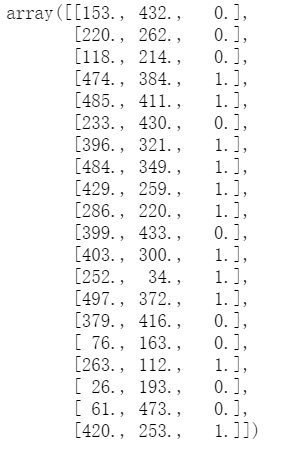二、逻辑回归实现

train_x = train[:,0:2]
train_y = train[:,2]
#初始化参数
theta = np.random.rand(3)
#标准化
mu = train_x.mean(axis = 0)
sigma = train_x.std(axis = 0)
def standardize(x):
return (x - mu)/ sigma
train_z = standardize(train_x)
#增加x0
def to_matrix(x):
x0 = np.ones([x.shape, 1])
return np.hstack([x0, x])
X = to_matrix(train_z)
#将标准化后的训练数据画成图
plt.plot(train_z[train_y == 1, 0], train_z[train_y == 1, 1], 'o')
plt.plot(train_z[train_y == 0, 0], train_z[train_y == 0, 1], 'x')
plt.show()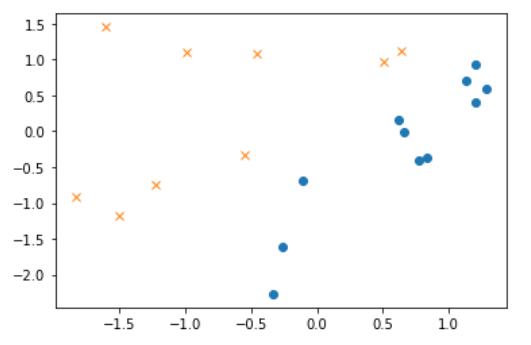预测函数

#sigmod函数
def f(x):
return 1 / (1 + np.exp(-np.dot(x, theta)))

到此事前准备就结束了，接下来是参数更新部分的实现。学习逻辑回归的时候，我们进行了定义逻辑回归的似然函数，对对数似然函数进行微分等一系列操作，然后最终得到的参数更新表达式是这样的。

与回归时一样，将当作向量来处理，将它与训练数据的矩阵相乘就行了，我们把重复次数设置得稍微多一点，比如5000 次左右。在实际问题中需要通过反复尝试来设置这个值，即通过确认学习中的精度来确定重复多少次才足够好。

#学习率
ETA = 1e-3
#重复次数
epoch = 5000
#重复学习
for _ in range(epoch):
theta = theta -ETA * np.dot(f(X) - train_y, X)

那我们用图来确认一下结果吧。之前说过在逻辑回归中，这条直线是决策边界。 也就是说，时图像是横向的，时图像是纵向的。将 变形并加以整理，得到这样的表达式。我们把它用图来展示一下看看。

x0 = np.linspace(-2, 2, 100)
plt.plot(train_z[train_y == 1, 0], train_z[train_y == 1, 1], 'o')
plt.plot(train_z[train_y == 0, 0], train_z[train_y == 0, 1], 'x')
plt.plot(x0, -(theta + theta * x0) / theta, linestyle = 'dashed')
plt.show()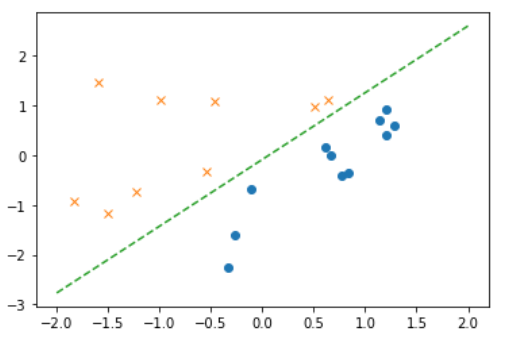上图可发现通过逻辑回归也能很好地分类了

三、验证

接下来我们尝试对任意图像进行分类。不要忘了对预测数据进行标准化哦。

f(to_matrix(standardize([[200, 100],[100, 200]])))

输出结果：array([0.91677595, 0.0297916 ])

所以前面200 × 100 的图像对应的值0.917 403 19 意味着图像是横向的概率为91.7%，而100 × 200 的图像对应的值0.029 557 52 意味着图像是纵向的概率为2.9%。

直接看概率可能不够直观，我们可以确定一个阈值，然后定义一个根据阈值返回1 或0 的函数。

def classify(x):
return (f(x) >= 0.5).astype(np.int)
classify(to_matrix(standardize([[200, 100], [100, 200]])))

输出结果：array([1, 0])

200×100 被分类为横向，而100×200 被分类为纵向了。

以上代码汇总

import numpy as np
import matplotlib.pyplot as plt
#读入训练数据
train_x = train[:,0:2]
train_y = train[:,2]
#初始化参数
theta = np.random.rand(3)
#标准化
mu = train_x.mean(axis = 0)
sigma = train_x.std(axis = 0)
def standardize(x):
return (x - mu)/ sigma
train_z = standardize(train_x)
#增加x0
def to_matrix(x):
x0 = np.ones([x.shape, 1])
return np.hstack([x0, x])
X = to_matrix(train_z)
#sigmod函数
def f(x):
return 1 / (1 + np.exp(-np.dot(x, theta)))
#分类函数
def classify(x):
return (f(x) >= 0.5).astype(np.int)
#学习率
ETA = 1e-3
#重复次数
count = 0
for _ in range(epoch):
theta = theta - ETA * np.dot(f(X) - train_y, X)
# 日志输出
count += 1
print(' 第{} 次: theta = {}'.format(count, theta))

#绘图确认
x0 = np.linspace(-2, 2, 100)
plt.plot(train_z[train_y == 1, 0], train_z[train_y == 1, 1], 'o')
plt.plot(train_z[train_y == 0, 0], train_z[train_y == 0, 1], 'x')
plt.plot(x0, -(theta + theta * x0) / theta, linestyle='dashed')
plt.show()

训练结果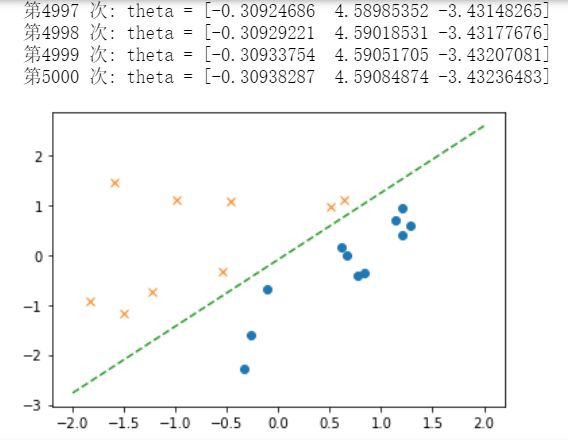四、线性不可分分类的实现

查看数据分布

import numpy as np
import matplotlib.pyplot as plt
#读入训练数据
train_x = train[:,0:2]
train_y = train[:, 2]
plt.plot(train_x[train_y == 1, 0], train_x[train_y == 1, 1], 'o')
plt.plot(train_x[train_y == 0, 0], train_x[train_y == 0, 1], 'x')
plt.show()

在图像上显示分布情况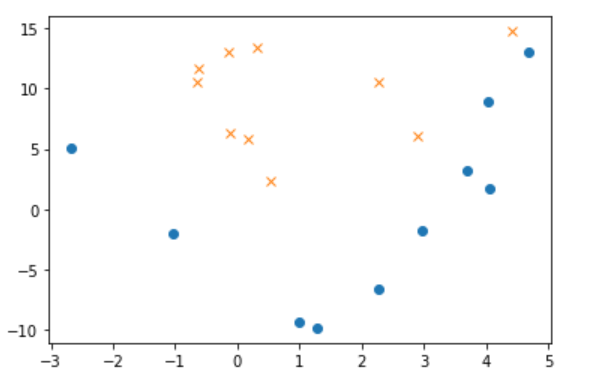我们试着用二次函数，即在训练数据里加上尝试分类，也就是说要增加一个 参数，参数总数达到四个。

#参数初始化
theta = np.random.rand(4)
#标准化
mu = train_x.mean(axis = 0)
sigma = train_x.std(axis = 0)
def standardize(x):
return (x - mu) / sigma
train_z = standardize(train_z)
#增加x0和x0
def to_matrix(x):
x0 = np.ones([x.shape, 1])
x3 = x[:,0,np.newaxis] ** 2
return np.hstack([x0, x, x3])#增加一个维度为x平方
X = to_matrix(train_z)

sigmoid 函数和学习的部分与刚才完全一样就行，可以直接执行了。

#sigmod函数
def f(x):
return 1 / (1 + np.exp(-np.dot(x, theta)))
#学习率
ETA = 1e-3
#重复次数
epoch = 5000
#重复学习
for _ in range(epoch):
theta = theta - ETA * np.dot(f(X) - train_y, X)
#日志输出
count += 1
print('第{}次:theta = {}'.format(count, theta))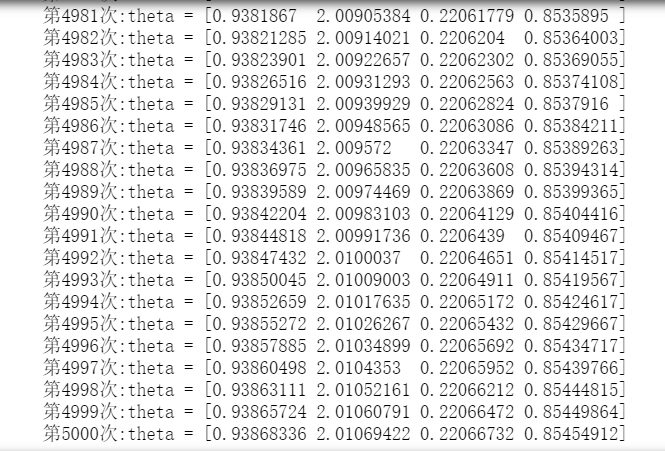对于有四个参数的可以这样变形，然后按这个公式画图就行了。

x1= np.linspace(-2, 2, 100)
x2 = -(theta + theta * x1 + theta * x1 ** 2) / theta
plt.plot(train_z[train_y == 1, 0], train_z[train_y == 1, 1], 'o')
plt.plot(train_z[train_y == 0, 0], train_z[train_y == 0, 1], 'x')
plt.plot(x1, x2, linestyle = 'dashed')
plt.show()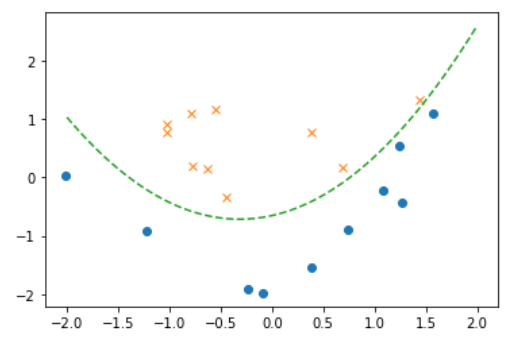如上图，决策边界已经变成曲线了。和回归时一样，将重复次数作为横轴、精度作为纵轴来绘图，这次应该会看到精度上升的样子。

精度的计算公式如下：

我们来验证一下

#参数初始化
theta = np.random.rand(4)
#精度的历史记录
accuracies = []
#重复学习
for _ in range(epoch):
theta = theta -ETA * np.dot(f(X) - train_y, X)
#计算现在的精度
result = classify(X) == train_y
accuracy = len(result[result == True]) / len(result)
accuracies.append(accuracy)
#将精度画成图
x = np.arange(len(accuracies))

plt.plot(x, accuracies)
plt.show()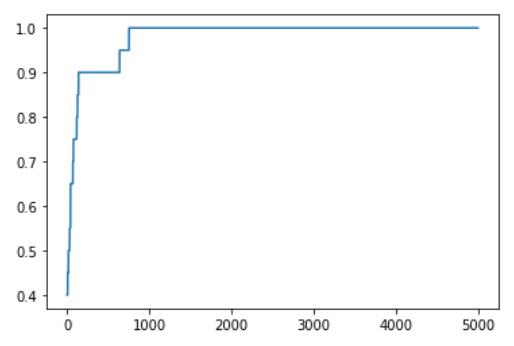如上图，随着次数的增加，精度的确变好了。这是训练数据只有20 个的缘故，精度值只能为0.05 的整数倍，所以这条线看起来有棱有角。而且从图中可以看出，在重复满5000 次之前，精度已经到1.0 了。刚才我是随口说了个5000 次，也可以像这样，每次学习后都计算精度，当精度达到满意的程度后就停止学习。

五、随机梯度下降算法的实现

我们再像回归时所做的那样，试试随机梯度下降法的实现，要做的也就是把学习部分稍稍修改一下。

#参数初始化
theta = np.random.rand(4)
#重复学习
for _ in range(epoch):
p = np.random.permutation(X.shape)#numpy.random.permutation(length)用来产生一个随机序列作为索引，再使用这个序列从原来的数据集中按照新的随机顺序产生随机数据集。length 为训练数据的个数。
for x, y in zip(X[p,:], train_y[p]):
theta = theta - ETA * (f(x) - y) * x
x1= np.linspace(-2, 2, 100)#在闭区间[-2, 2]生成200个均匀间隔的数字
x2 = -(theta + theta * x1 + theta * x1 ** 2) / theta
plt.plot(train_z[train_y == 1, 0], train_z[train_y == 1, 1], 'o')
plt.plot(train_z[train_y == 0, 0], train_z[train_y == 0, 1], 'x')
plt.plot(x1, x2, linestyle = 'dashed')
plt.show()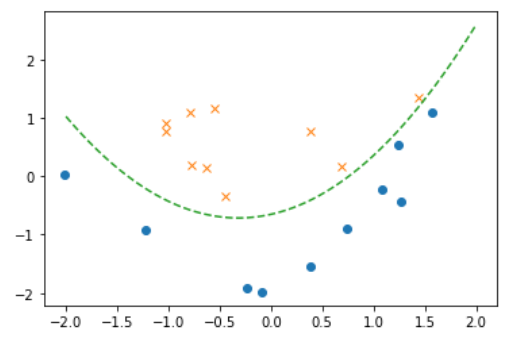从上图可以发现，分类的效果很不错！

展开全文weixin_43734080 2021-07-19 21:22:07
• 一、逻辑回归(LogisticRegression)Logistic regression (逻辑回归)是当前业界比较常用的机器学习方法，用于估计某种事物的可能性。还有类似的某用户购买某商品的可能性，某病人患有某种疾病的可能性啊等等。这个世界...

一、逻辑回归(LogisticRegression)

Logistic regression (逻辑回归)是当前业界比较常用的机器学习方法，用于估计某种事物的可能性。

还有类似的某用户购买某商品的可能性，某病人患有某种疾病的可能性啊等等。这个世界是随机的(当然了，人为的确定性系统除外，但也有可能有噪声或产生错 误的结果，只是这个错误发生的可能性太小了，小到千万年不遇，小到忽略不计而已)，所以万物的发生都可以用可能性或者几率(Odds)来表达。“几率”指 的是某事物发生的可能性与不发生的可能性的比值。

Logistic regression可以用来回归，也可以用来分类，主要是二分类。还记得上几节讲的支持向量机SVM吗？它就是个二分类的例如，它可以将两个不同类别的 样本给分开，思想是找到最能区分它们的那个分类超平面。但当你给一个新的样本给它，它能够给你的只有一个答案，你这个样本是正类还是负类。例如你问 SVM，某个女生是否喜欢你，它只会回答你喜欢或者不喜欢。这对我们来说，显得太粗鲁了，要不希望，要不绝望，这都不利于身心健康。那如果它可以告诉我， 她很喜欢、有一点喜欢、不怎么喜欢或者一点都不喜欢，你想都不用想了等等，告诉你她有49%的几率喜欢你，总比直接说她不喜欢你，来得温柔。而且还提供了 额外的信息，她来到你的身边你有多少希望，你得再努力多少倍，知己知彼百战百胜，哈哈。Logistic regression就是这么温柔的，它给我们提供的就是你的这个样本属于正类的可能性是多少。

还得来点数学。(更多的理解，请参阅参考文献)假设我们的样本是{x, y}，y是0或者1，表示正类或者负类，x是我们的m维的样本特征向量。那么这个样本x属于正类，也就是y=1的“概率”可以通过下面的逻辑函数来表示：这里θ是模型参数，也就是回归系数，σ是sigmoid函数。实际上这个函数是由下面的对数几率(也就是x属于正类的可能性和负类的可能性的比值的对数)变换得到的：换句话说，y也就是我们关系的变量，例如她喜不喜欢你，与多个自变量(因素)有关，例如你人品怎样、车子是两个轮的还是四个轮的、长得胜过潘安还是和犀利哥有得一拼、有千尺豪宅还是三寸茅庐等等，我们把这些因素表示为x1, x2,…, xm。 那这个女的怎样考量这些因素呢？最快的方式就是把这些因素的得分都加起来，最后得到的和越大，就表示越喜欢。但每个人心里其实都有一杆称，每个人考虑的因 素不同，萝卜青菜，各有所爱嘛。例如这个女生更看中你的人品，人品的权值是0.6，不看重你有没有钱，没钱了一起努力奋斗，那么有没有钱的权值是 0.001等等。我们将这些对应x1, x2,…, xm的权值叫做回归系数，表达为θ1, θ2,…, θm。他们的加权和就是你的总得分了。请选择你的心仪男生，非诚勿扰！哈哈。

所以说上面的logistic回归就是一个线性分类模型，它与线性回归的不同点在于：为了将线性回归输出的很大范围的数，例如从负无穷到正无穷，压缩到 0和1之间，这样的输出值表达为“可能性”才能说服广大民众。当然了，把大值压缩到这个范围还有个很好的好处，就是可以消除特别冒尖的变量的影响(不知道 理解的是否正确)。而实现这个伟大的功能其实就只需要平凡一举，也就是在输出加一个logistic函数。另外，对于二分类来说，可以简单的认为：如果样 本x属于正类的概率大于0.5，那么就判定它是正类，否则就是负类。实际上，SVM的类概率就是样本到边界的距离，这个活实际上就让logistic regression给干了。所以说，LogisticRegression 就是一个被logistic方程归一化后的线性回归，仅此而已。

好了，关于LR的八卦就聊到这。归入到正统的机器学习框架下，模型选好了，只是模型的参数θ还是未知的，我们需要用我们收集到的数据来训练求解得到它。那我们下一步要做的事情就是建立代价函数了。

LogisticRegression最基本的学习算法是最大似然。啥叫最大似然，可以看看我的另一篇博文“从最大似然到EM算法浅解”。

假设我们有n个独立的训练样本{(x1, y1) ,(x2, y2),…, (xn, yn)}，y={0, 1}。那每一个观察到的样本(xi, yi)出现的概率是：面为什么是这样呢？当y=1的时候，后面那一项是不是没有了，那就只剩下x属于1类的概率，当y=0的时候，第一项是不是没有了，那就只剩下后面那个x属

于0的概率(1减去x属于1的概率)。所以不管y是0还是1，上面得到的数，都是(x,

y)出现的概率。那我们的整个样本集，也就是n个独立的样本出现的似然函数为(因为每个样本都是独立的，所以n个样本出现的概率就是他们各自出现的概率相

乘)：那最大似然法就是求模型中使得似然函数最大的系数取值θ*。这个最大似然就是我们的代价函数(cost function)了。

OK，那代价函数有了，我们下一步要做的就是优化求解了。我们先尝试对上面的代价函数求导，看导数为0的时候可不可以解出来，也就是有没有解析解，有这个解的时候，就皆大欢喜了，一步到位。如果没有就需要通过迭代了，耗时耗力。

我们先变换下L(θ)：取自然对数，然后化简(不要看到一堆公式就害怕哦，很简单的哦，只需要耐心一点点，自己动手推推就知道了。注：有xi的时候，表示它是第i个样本，下面没有做区分了，相信你的眼睛是雪亮的)，得到：这时候，用L(θ)对θ求导，得到：然后我们令该导数为0，你会很失望的发现，它无法解析求解。不信你就去尝试一下。所以没办法了，只能借助高大上的迭代来搞定了。这里选用了经典的梯度下降算法。

二、优化求解

Gradient descent 又叫 steepest descent，是利用一阶的梯度信息找到函数局部最优解的一种方法，也是机器学习里面最简单最常用的一种优化方法。它的思想很简单，和我开篇说的那样， 要找最小值，我只需要每一步都往下走(也就是每一步都可以让代价函数小一点)，然后不断的走，那肯定能走到最小值的地方，例如下图所示：但，我同时也需要更快的到达最小值啊，怎么办呢？我们需要每一步都找下坡最快的地方，也就是每一步我走某个方向，都比走其他方法，要离最小值更近。而这个下坡最快的方向，就是梯度的负方向了。

对logistic Regression来说，梯度下降算法新鲜出炉，如下：其中，参数α叫学习率，就是每一步走多远，这个参数蛮关键的。如果设置的太多，那么很容易就在最优值附加徘徊，因为你步伐太大了。例如要从广州到上海，但 是你的一步的距离就是广州到北京那么远，没有半步的说法，自己能迈那么大步，是幸运呢？还是不幸呢？事物总有两面性嘛，它带来的好处是能很快的从远离最优 值的地方回到最优值附近，只是在最优值附近的时候，它有心无力了。但如果设置的太小，那收敛速度就太慢了，向蜗牛一样，虽然会落在最优的点，但是这速度如 果是猴年马月，我们也没这耐心啊。所以有的改进就是在这个学习率这个地方下刀子的。我开始迭代是，学习率大，慢慢的接近最优值的时候，我的学习率变小就可 以了。所谓采两者之精华啊！这个优化具体见2.3 。

梯度下降算法的伪代码如下：

################################################

初始化回归系数为1

重复下面步骤直到收敛{

计算整个数据集的梯度

}

返回回归系数值

################################################

注：因为本文中是求解的Logit回归的代价函数是似然函数，需要最大化似然函数。所以我们要用的是梯度上升算法。但因为其和梯度下降的原理是一样的，只 是一个是找最大值，一个是找最小值。找最大值的方向就是梯度的方向，最小值的方向就是梯度的负方向。不影响我们的说明，所以当时自己就忘了改过来了，谢谢 评论下面@wxltt的指出。另外，最大似然可以通过取负对数，转化为求最小值。代码里面的注释也是有误的，写的代码是梯度上升，注销成了梯度下降，对大 家造成的不便，希望大家海涵。

梯度下降算法在每次更新回归系数的时候都需要遍历整个数据集(计算整个数据集的回归误差)，该方法对小数据集尚可。但当遇到有数十亿样本和成千上万的特征 时，就有点力不从心了，它的计算复杂度太高。改进的方法是一次仅用一个样本点(的回归误差)来更新回归系数。这个方法叫随机梯度下降算法。由于可以在新的 样本到来的时候对分类器进行增量的更新(假设我们已经在数据库A上训练好一个分类器h了，那新来一个样本x。对非增量学习算法来说，我们需要把x和数据库 A混在一起，组成新的数据库B，再重新训练新的分类器。但对增量学习算法，我们只需要用新样本x来更新已有分类器h的参数即可)，所以它属于在线学习算 法。与在线学习相对应，一次处理整个数据集的叫“批处理”。

随机梯度下降算法的伪代码如下：

################################################

初始化回归系数为1

重复下面步骤直到收敛{

对数据集中每个样本

计算该样本的梯度

}

返回回归系数值

################################################

2.3、改进的随机梯度下降

评价一个优化算法的优劣主要是看它是否收敛，也就是说参数是否达到稳定值，是否还会不断的变化？收敛速度是否快？上图展示了随机梯度下降算法在200次迭代中(请先看第三和第四节再回来看这里。我们的数据库有100个二维样本，每个样本都对系数调整一次，所以共有

200*100=20000次调整)三个回归系数的变化过程。其中系数X2经过50次迭代就达到了稳定值。但系数X1和X0到100次迭代后稳定。而且可

恨的是系数X1和X2还在很调皮的周期波动，迭代次数很大了，心还停不下来。产生这个现象的原因是存在一些无法正确分类的样本点，也就是我们的数据集并非

线性可分，但我们的logistic

regression是线性分类模型，对非线性可分情况无能为力。然而我们的优化程序并没能意识到这些不正常的样本点，还一视同仁的对待，调整系数去减少

对这些样本的分类误差，从而导致了在每次迭代时引发系数的剧烈改变。对我们来说，我们期待算法能避免来回波动，从而快速稳定和收敛到某个值。

对随机梯度下降算法，我们做两处改进来避免上述的波动问题：

1)

在每次迭代时，调整更新步长alpha的值。随着迭代的进行，alpha越来越小，这会缓解系数的高频波动(也就是每次迭代系数改变得太大，跳的跨度太

大)。当然了，为了避免alpha随着迭代不断减小到接近于0(这时候，系数几乎没有调整，那么迭代也没有意义了)，我们约束alpha一定大于一个稍微

大点的常数项，具体见代码。

2)每次迭代，改变样本的优化顺序。也就是随机选择样本来更新回归系数。这样做可以减少周期性的波动，因为样本顺序的改变，使得每次迭代不再形成周期性。

改进的随机梯度下降算法的伪代码如下：

################################################

初始化回归系数为1

重复下面步骤直到收敛{

对随机遍历的数据集中的每个样本

随着迭代的逐渐进行，减小alpha的值

计算该样本的梯度

}

返回回归系数值

################################################比较原始的随机梯度下降和改进后的梯度下降，可以看到两点不同：

1)系数不再出现周期性波动。2)系数可以很快的稳定下来，也就是快速收敛。这里只迭代了20次就收敛了。而上面的随机梯度下降需要迭代200次才能稳定。

三、Python实现

#-*- coding: utf-8 -*-

"""Created on Mon Dec 29 15:16:40 2014

from numpy import *

importmatplotlib.pyplot as pltimporttimedefsigmoid(inX):return 1.0 / (1 + exp(-inX))deftrainLogRegres(train_x, train_y, opts):

starttime=time.time()

numSamples, numFeatures=shape(train_x)printnumSamples,numFeatures

alpha= opts['alpha']; maxIter = opts['maxIter']

theta= ones((numFeatures, 1))#随机梯度下降

for k inrange(maxIter):for i inrange(numSamples):

output= sigmoid(train_x[i, :] *theta)

error= train_y[i, 0] -output

theta= theta + alpha * train_x[i, :].transpose() *error

endtime=time.time()print 'Training complete! Took %fs!' % (endtime -starttime)printthetareturnthetadeftestLogRegres(weights, test_x, test_y):

numSamples, numFeatures=shape(test_x)

matchCount=0for i inxrange(numSamples):

predict= sigmoid(test_x[i, :] *weights)if predict >= 0.5:

predict= 1

else:

predict=0if predict ==(test_y[i, 0]):

matchCount+= 1accuracy= float(matchCount) /numSamplesreturnaccuracydefshowLogRegres(weights, train_x, train_y):

numSamples, numFeatures=shape(train_x)#所有样本

for i inxrange(numSamples):if int(train_y[i, 0]) ==0:

plt.plot(train_x[i,1], train_x[i, 2], 'or')elif int(train_y[i, 0]) == 1:

plt.plot(train_x[i,1], train_x[i, 2], 'ob')#分类线

min_x = min(train_x[:, 1])[0, 0]

max_x= max(train_x[:, 1])[0, 0]

weights=weights.getA()

y_min_x= float(-weights - weights * min_x) / weights

y_max_x= float(-weights - weights * max_x) / weights

plt.plot([min_x, max_x], [y_min_x, y_max_x],'-g')

plt.xlabel('X1'); plt.ylabel('X2')

train_x=[]

train_y=[]

lineArr=line.strip().split()

train_x.append([1.0, float(lineArr), float(lineArr)])

test_x= train_x; test_y =train_yprint "step 2: training..."opts= {'alpha': 0.01, 'maxIter': 200}

optimalWeights=trainLogRegres(train_x, train_y, opts)print "step 3: testing..."accuracy=testLogRegres(optimalWeights, test_x, test_y)print "step 4: show the result..."

print 'The classify accuracy is: %.3f%%' % (accuracy * 100)

showLogRegres(optimalWeights, train_x, train_y)

展开全文weixin_39711348 2020-12-22 14:41:34
• weixin_32431435 2021-01-30 05:06:51
• weixin_32296949 2021-01-14 09:37:37
• weixin_33504929 2021-02-11 05:21:11
• weixin_34621309 2021-02-10 08:57:06
• weixin_34352633 2021-01-13 23:20:39
• weixin_39747755 2020-12-20 06:53:12
• weixin_33390343 2021-07-19 18:45:10
• 正则化逻辑回归-python实现 python 机器学习

CltCj 2021-08-29 15:17:41
• weixin_36023533 2020-12-30 14:20:01
• weixin_39531378 2020-12-21 14:31:13
• 神经网络思维的逻辑回归python代码 python 深度学习

weixin_44235615 2021-07-27 20:48:03
• u013946150 2021-01-10 10:52:25
• python实现逻辑回归预测 机器学习

qq_41606378 2021-04-06 01:27:57
• weixin_35056962 2021-02-03 12:17:18
• qq_23934063 2021-04-10 10:00:30
• 【python机器学习】逻辑回归算法实现（基于鸢尾花数据集） python 机器学习 逻辑回归

qq_42447107 2020-12-20 20:28:44
• weixin_35338559 2021-03-05 17:04:38
• weixin_35738304 2021-01-13 23:06:38
• weixin_35990295 2021-04-27 04:45:31
• weixin_39712969 2020-12-21 14:29:29
• weixin_42310670 2021-03-07 01:19:57
• m0_46882548 2021-02-02 11:26:02
• 逻辑回归（ROC、AUC、KS）-python实现-内含训练数据-测试数据 机器学习 深度学习 人工智能

woailaopoqq 2021-01-24 16:24:09
• sklearn:逻辑回归（ROC、AUC、KS）-python实现-内含训练数据-测试数据 python 机器学习 人工智能 数据挖掘

woailaopoqq 2021-01-24 00:37:52
• weixin_35427369 2020-12-30 13:49:56
• weixin_33274593 2021-02-11 05:21:27...

逻辑回归pythonpython 订阅